# 一.学习总结(2分)

### 1.1树结构思维导图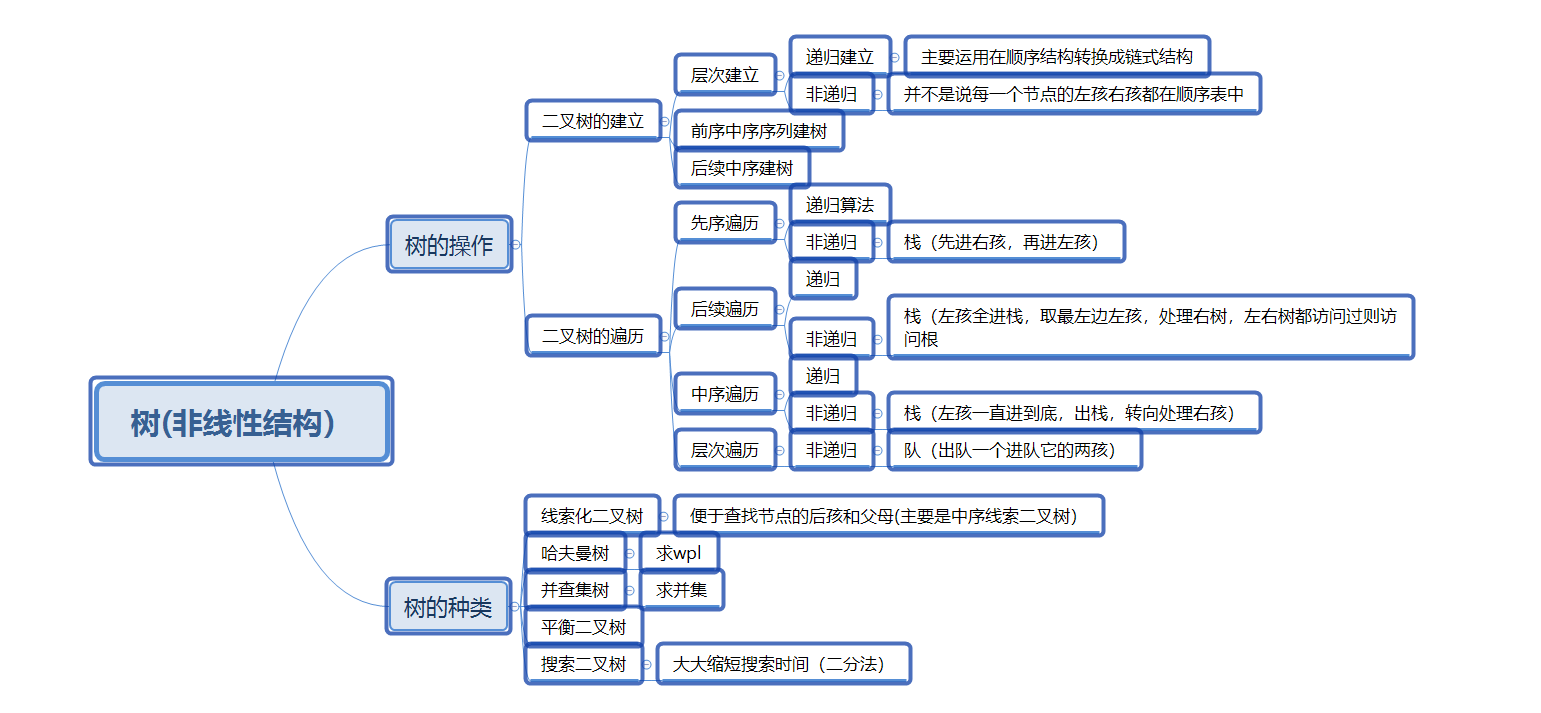### 1.2 树结构学习体会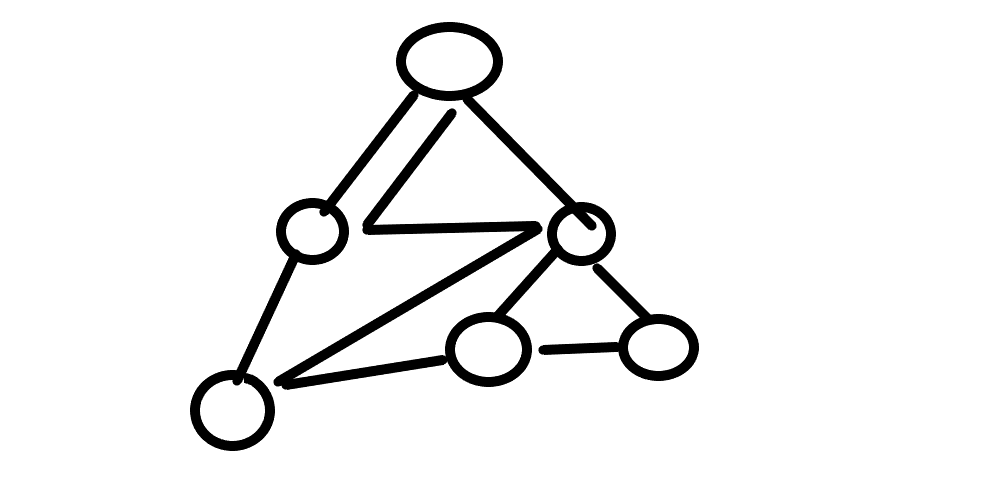while(队不空）
{

if(BT->lchild！=NULL）进队。
if(BT->rchild！=NULL）进队。
}
}

while(栈不空）{

if(BT->rchild！=NULL）进栈。
if(BT->lchild！=NULL）进栈。
}
}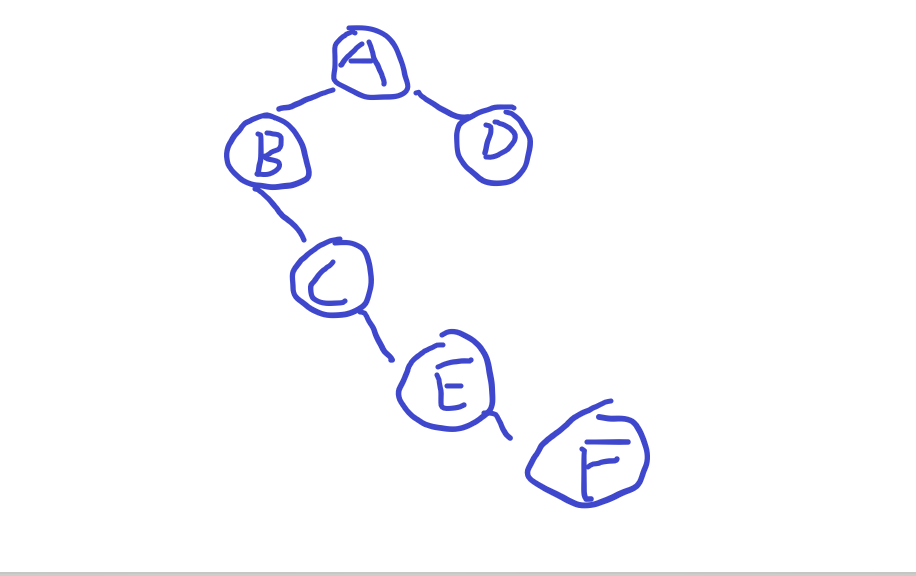a+b为第一个非根节点 则a+b+c为第二个依次类推

# 二.PTA实验作业（4分）

## 2.1 题目1：修理牧场

### 1 设计思路（伪代码或流程图）

定义一个队列可以让进队元素按从大到小排列
for(i=0;i<N;I++){

}
while(队不空）{

}



### 2.代码截图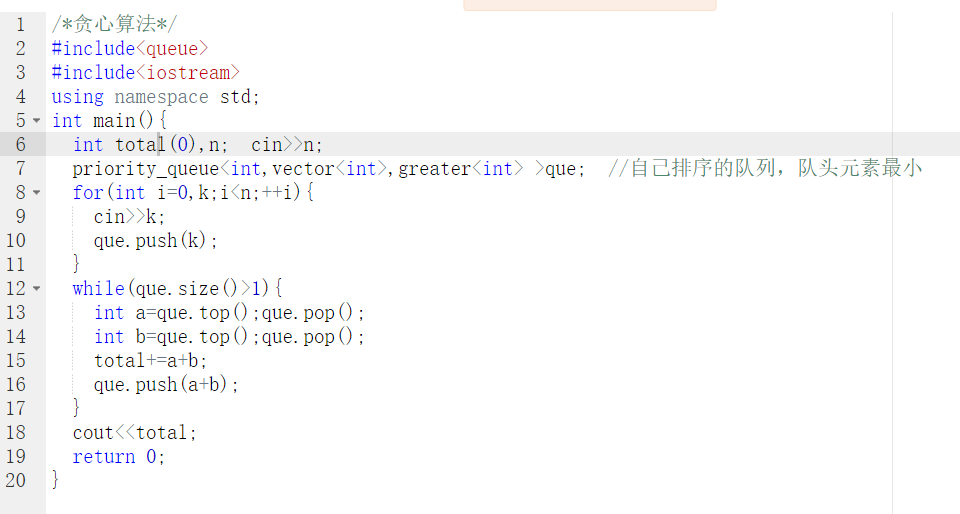### 3.PTA提交列表说明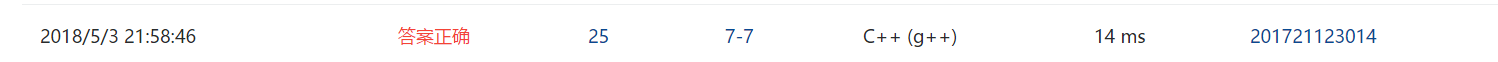## 2.1 题目2：朋友圈

### 1 设计思路（伪代码或流程图）

//定义三个数组一个是保留每一个每一个数对应的根节点一个保留所有根节点
//最后一个保留每一个根对应的孩子数

for(int i=0;i<m;i++){

}



### 2.代码截图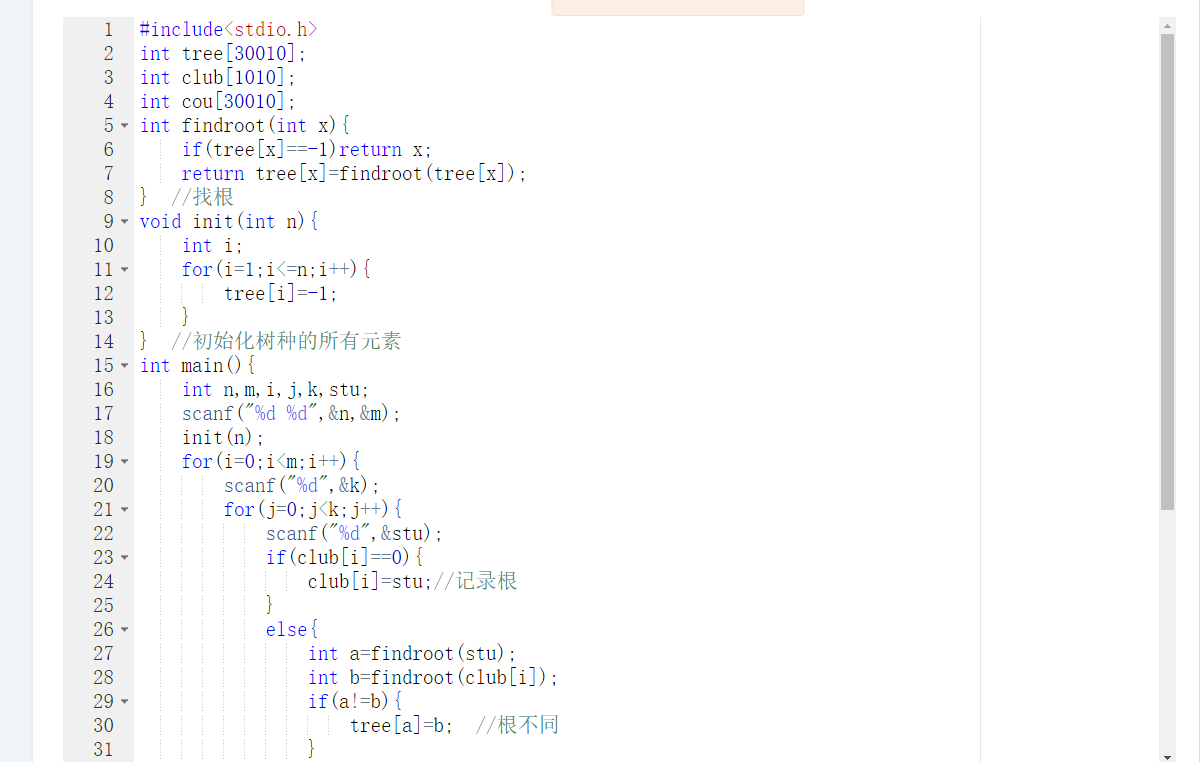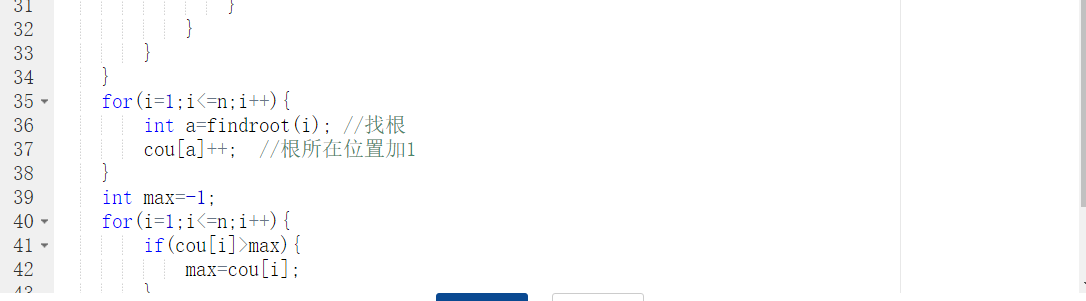### 3.PTA提交列表说明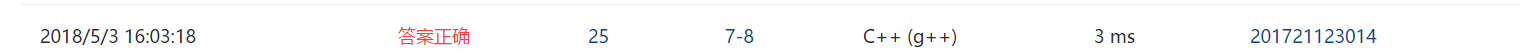## 2.1 题目3：表达式树

### 1 设计思路（伪代码或流程图）

//观察表达式树会发现数字字符的左孩子右孩子都是空的用于后面的表达式树的运算
//创建两个栈一个是树节点的保存类型一个是字符保存栈
for(int i=0;str[i];i++){
if（字符是数字）创建树节点并且入栈
else
{
if(字符栈栈顶优先级小于str[i]){

}
else if(字符栈栈顶优先级大于str[i]){

}
else{

}
}

{
if(BT->rchild==NULL&&BT->lchild==NULL)
return BT->data-'0'
else{
a=计算遍历右树
b=计算遍历左树
switch()
{
case '+':return a+b;
case  '-':return a-b
case '*':returna*b
case '/':return a/b
}
}



### 2.代码截图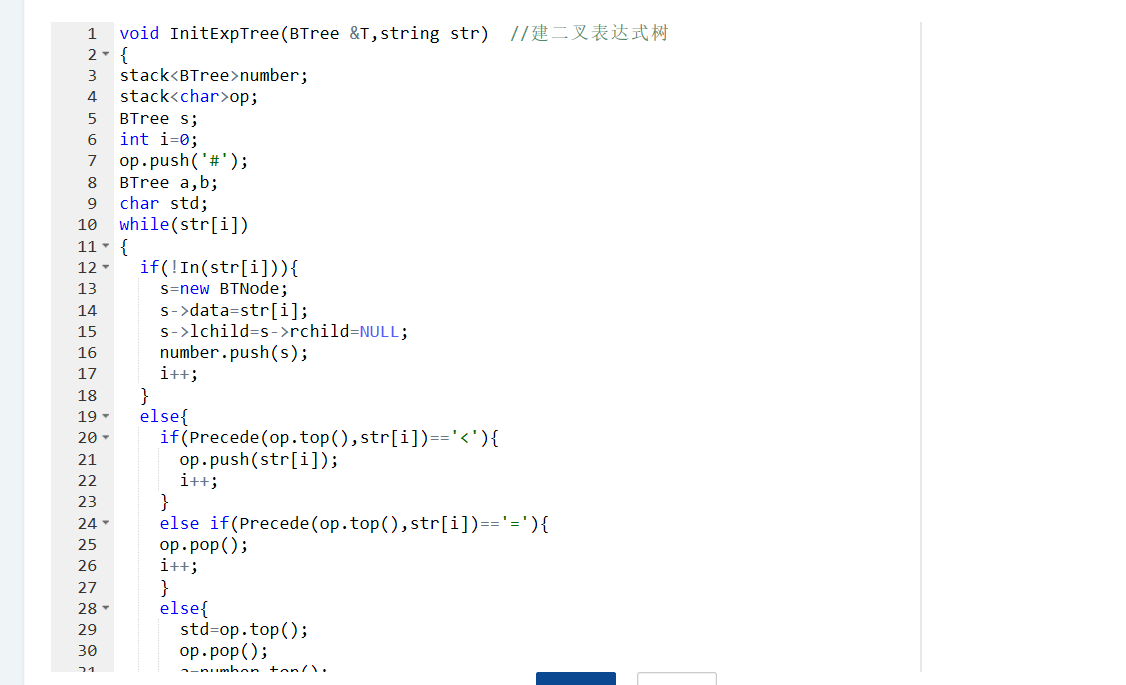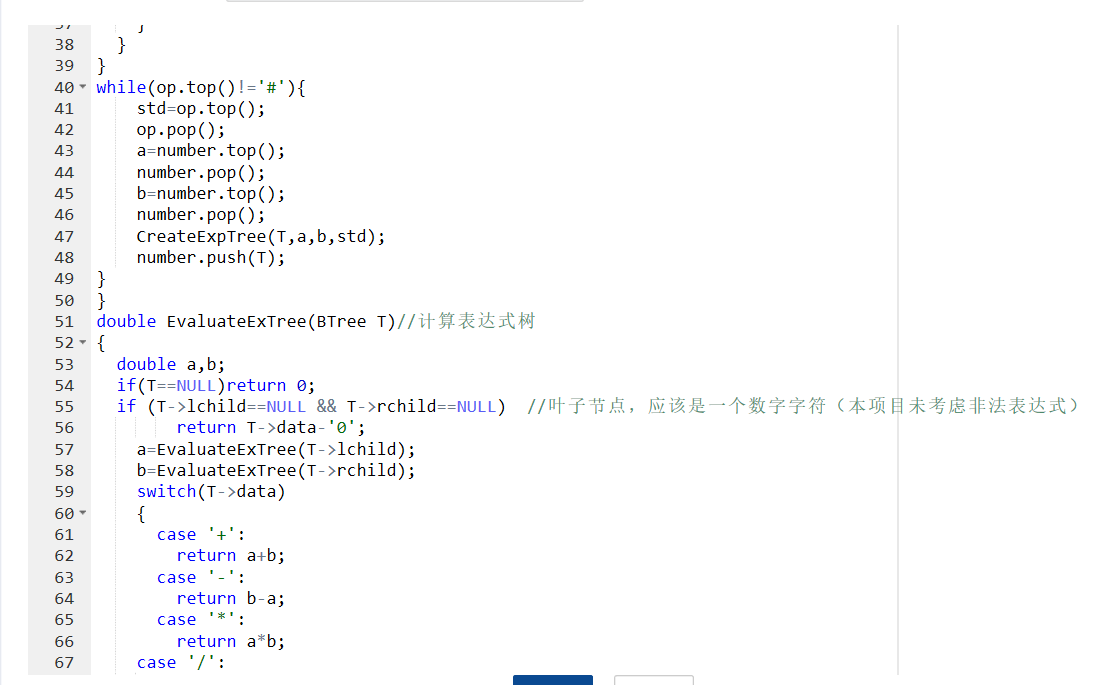### 3.PTA提交列表说明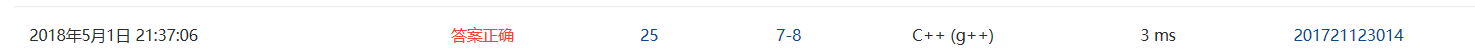## 3.3 我的总分：230# 四. 阅读代码（必做，1分）

5-27 家谱处理 (30分)

#include <stdio.h>
#include<stdlib.h>
#include<string.h>
/* 评测结果 时间  结果  得分  题目  编译器     用时（ms）  内存（MB）  用户
2016-08-30 10:31    全部正确    25  5-27    gcc     1   1   569985011

typedef struct node *Node;
struct node {
char Name;
int space;
int  Parant;
};

Node Tree;
int n;

int Scan(char*);
int Trace(int);
int judgeParent(int,int);//父子
int judgeSibling(int,int);//兄弟
int judgeAncestor(int,int);//祖先
void work();
int Index(char*);

int main() {
int m;
scanf("%d%d",&n,&m);
Tree=(Node)malloc(sizeof(struct node)*n);
getchar();//清除缓存
for(int i=0; i<n; i++) {
Tree[i].space=Scan(Tree[i].Name);
Tree[i].Parant=i;
}
Tree.Parant=-1;

for(int i=0; i<m; i++) {
work();
getchar();
}

return 0;
}
int judgeParent(int x,int y) {
if(Tree[x].Parant==x)Tree[x].Parant=Trace(x);
return Tree[x].Parant==y;
}
int judgeSibling(int x,int y) {
if(Tree[x].Parant==x)Tree[x].Parant=Trace(x);
if(Tree[y].Parant==y)Tree[y].Parant=Trace(y);
return Tree[x].Parant==Tree[y].Parant;
}
int judgeAncestor(int x,int y) {
while(x!=-1) {
if(judgeParent(x,y))return 1;
else x=Tree[x].Parant;
}
return 0;
}

void work() {
char StrX,StrY,relation;
scanf("%s%*s%*s%s%*s%s",StrX,relation,StrY);
//  printf("%s - %s - %s\n",StrX,relation,StrY);

int X=Index(StrX);
int Y=Index(StrY);
//  printf("%d   -    %d",X,Y);
int result;
switch(relation) {
case 'c':
result=judgeParent(X,Y);
break;
case 'p':
result=judgeParent(Y,X);
break;
case 's':
result=judgeSibling(X,Y);
break;
case 'd':
result=judgeAncestor(X,Y);
break;
case 'a':
result=judgeAncestor(Y,X);
break;
default:
result=-1;
break;
}

if(result==1)printf("True\n");
else if(!result)printf("False\n");
//  else printf("ERROR:系统不能识别所指定关系！\n");
}

int Index(char*a) {
for(int i=0; i<n; i++) {
//      printf("*");
if(strcmp(Tree[i].Name,a)==0)return i;
}
//  printf("ERROR:所给人名不存在！\n");
return -1;
}

int Trace(int child) { //往前遍历第一个比他缩进少的就是他的父亲

for(int i=child-1; i>=0; i--) {
if(Tree[i].space<Tree[child].space) {
//      printf("%d's parent is %d'",child,i);
return i;
}
}
return -1;//如果没有，那么他就是亚当夏娃了。
}

int Scan(char*p) {
char c;
int space=0;

while((c=getchar())==' ')space++;//记录字符串前面的空格数量

do {
*p++=c;
} while((c=getchar())!='\n');
*p='\0';

return space;
}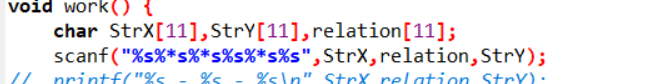# 五. 代码Git提交记录截图posted @ 2018-05-05 17:43  这个人有毒啊  阅读(256)  评论(0编辑  收藏  举报